# TensorFlow应用实战-5- TensorFlow基础知识

## 从helloworld开始

mkdir 1.helloworld
cd 1.helloworld

vim helloworld.py


# -*- coding: UTF-8 -*-

# 引入 TensorFlow 库
import tensorflow as tf

# 设置了gpu加速提示信息太多了，设置日志等级屏蔽一些
import os
os.environ['TF_CPP_MIN_LOG_LEVEL']='3'

# 创建一个常量 Operation (操作)
hw = tf.constant("Hello World! Mtianyan love TensorFlow!")

# 启动一个 TensorFlow 的 Session (会话)
sess = tf.Session()

# 运行 Graph (计算图)
print (sess.run(hw))

# 关闭 Session（会话）
sess.close()


## TensorFlow的编程模式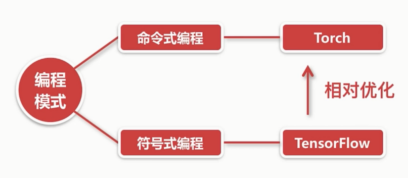mark

• 容易理解，命令语句基本没优化: C,java, C++, Python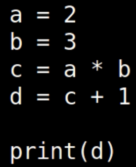mark

• 涉及较多的嵌入和优化，运行速度有同比提升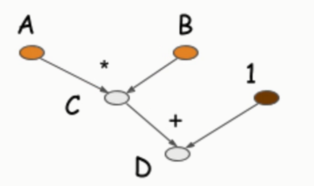mark

# -*- coding: UTF-8 -*-

# 引入 TensorFlow 库
import tensorflow as tf

# 设置了gpu加速提示信息太多了，设置日志等级屏蔽一些
import os
os.environ['TF_CPP_MIN_LOG_LEVEL']='3'

a = tf.constant(2)
b = tf.constant(3)
c = tf.multiply(a,b)

with tf.Session() as sess:
print (sess.run(d))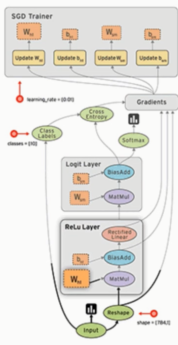mark

TensorFlow的计算流图，符号式编程的范式。有节点有边，边是计算结果在节点中流动。

## TensorFlow的基础结构

Tensor 在 计算流图中流动(flow)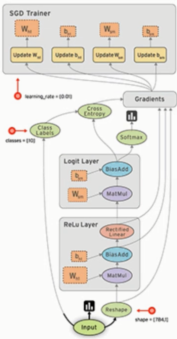mark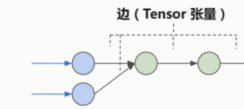mark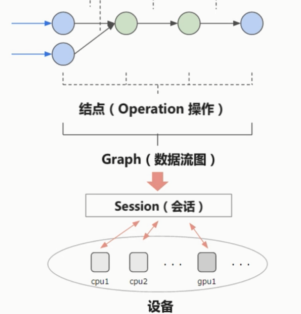mark

### 图的基本构成

• Tensor (张量) 边里流动的数据
• Operation(操作)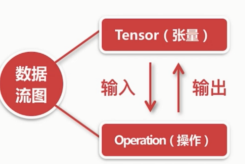mark

Tensor 会作为operation的输入，operation输出的依然是Tensor

### TensorFlow的基础模型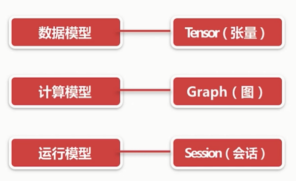mark

## 图(Graph)与会话(Session)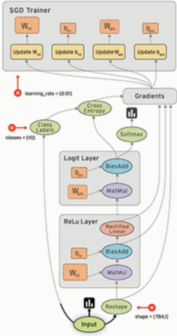mark

### 数据流图的结构markmark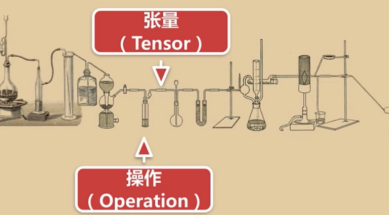mark

### 什么是会话(Session)？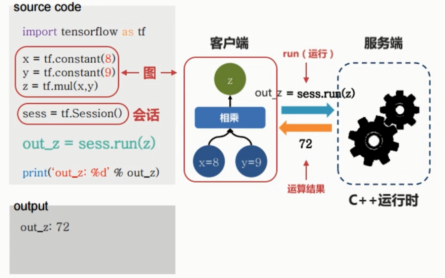mark

TensorFlow使用了客户端和服务端的经典架构。

### Session的作用mark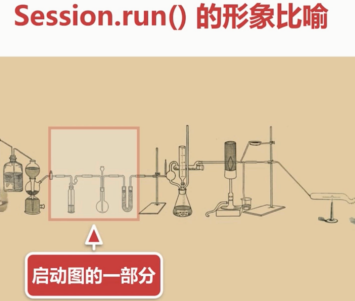mark

### TensorFlow程序的流程

1. 定义算法的计算图(Graph)的结构 静态
2. 使用会话(Session) 执行计算

## python常用库numpy

TensorFlow和numpy有一定联系

• 有很多类似的概念和api

numpy官网，科学计算。n阶数组对象。

numpy速度是非常快的，比原生快很多。

scipy 是一个开源软件。Matplotlib。pandas。jupyter notebook

numpy的操作对象是一个多维的数组。类似Tensor

ndarray ndim shape size dtype(同一类型元素)

import numpy as np
vector = np.array([1,2,3])
vector.shape
vector.size
vector.ndim
type(vector)

# 创建二维数组(矩阵)
matrix = np.array([[1, 2],[3, 4]])
matrix.shape
matrix.size
matrix.ndim
type(matrix)


one = np.arange(12)
# 0 - 11
one.reshape((3,4))
two = one.reshape((3,4))

two.shape
two.size
two.ndim


## 什么是Tensor(张量)?mark

TensorFlow里的数据都是Tensor，所以它可以说是一个张量的流图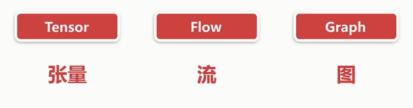mark

### 张量的维度(秩): Rank / Order dimension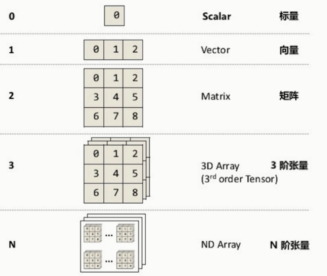mark

vector & Matrix

numpy中的基础要素就是array，和Tensor 差不多的一种表述。

import numpy as np
zeros = np.zeros((3,4))
zeros

ones = np.ones((5,6))
ones

# 对角矩阵: 必须是一个方阵.对角线是1，其他都是0的方阵
ident = np.eye(4)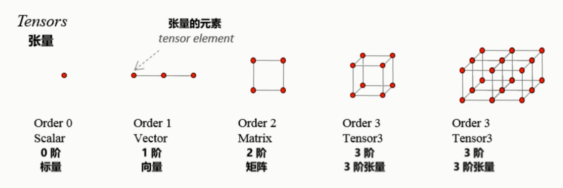markmark

### Tensor 的属性

TensorFlow.datatype list

https://www.tensorflow.org/api_docs/python/tf/DType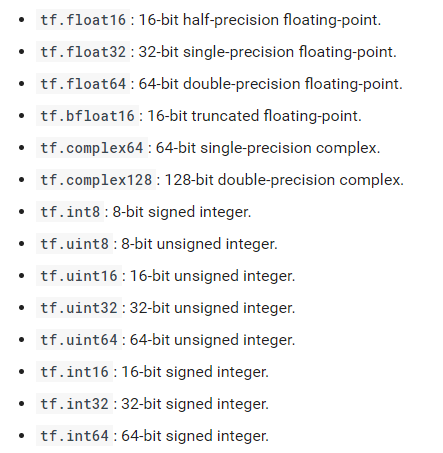mark

TensorFlow数据类型有很多。

https://www.tensorflow.org/api_docs/python/tf/Tensor

A Tensor是一个输出的符号句柄 Operation。它不包含该操作输出的值，而是提供了在TensorFlow中计算这些值的方法tf.Session。

device，在哪个设备上被计算出来的。

Graph 这个Tensor 所属的一个图

name 是我们可以给张量起的名字

op 是产生这个Tensor 的一个操作

• Constant
• Variable
• Placeholder
• SparseTensor

### Constant (常量)

• 值不能改变的一种Tensor

tf.constant(
value,
dtype=None,
shape=None,
name='Const',
verify_shape=False
)


verify_shape 验证形状

# Constant 1-D Tensor populated with value list.
tensor = tf.constant([1, 2, 3, 4, 5, 6, 7]) => [1 2 3 4 5 6 7]

# Constant 2-D tensor populated with scalar value -1.
tensor = tf.constant(-1.0, shape=[2, 3]) => [[-1. -1. -1.]
[-1. -1. -1.]]


const = tf.constant(3)
const
# 输出const：0 shape=() dtype=int32


run之后才能得到具体的数。与普通的变量常量是不一样的。

### Variable 变量

• 值可以改变的一种tensor

__init__(
initial_value=None,
trainable=True,
collections=None,
validate_shape=True,
caching_device=None,
name=None,
variable_def=None,
dtype=None,
expected_shape=None,
import_scope=None,
constraint=None
)


var = tf.Variable(3)
var
# 不会输出真实值，只会输出数据类型等特征量


var1 = tf.Variable(4, dtype=tf.int64)
var1
# 默认系统给的变量名会自动递增


### PlaceHolder(占位符)

• 先占住一个固定的位置，等着你之后往里面添加值的一种Tensormark

tf.placeholder

https://www.tensorflow.org/api_docs/python/tf/placeholder

tf.placeholder(
dtype,
shape=None,
name=None
)


x = tf.placeholder(tf.float32, shape=(1024, 1024))
y = tf.matmul(x, x)

with tf.Session() as sess:
print(sess.run(y))  # ERROR: will fail because x was not fed.

rand_array = np.random.rand(1024, 1024)
print(sess.run(y, feed_dict={x: rand_array}))  # Will succeed.


feed_dict 真正运行时才通过feed_dict关键字以字典形式向里面传值。

### SparseTensor(稀疏张量)

• 一种"稀疏"的Tensor，类似线性代数里面的稀疏矩阵的概念

tf.SparseTensor

SparseTensor(indices=[[0, 0], [1, 2]], values=[1, 2], dense_shape=[3, 4])


[[1, 0, 0, 0]
[0, 0, 2, 0]
[0, 0, 0, 0]]


### Tensor 表示法

Tensor("MUL:0", shape=(),dtype=float32)


0表示索引

shape 就是形状 dtype 数据类型

named_var = tf.Variable([5,6], name="named_var")
named_var


## 图和会话原理及案例

Graph(图)的形象比喻mark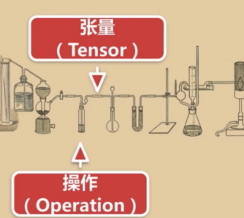mark

### TensorFlow程序的流程

1. 定义算法的计算图(Graph)结构

1. 使用会话(Session)执行图的一部分(计算)

Graph tf.Graph

https://www.tensorflow.org/api_docs/python/tf/Graph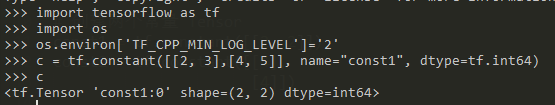mark

https://www.tensorflow.org/api_docs/python/tf/Session

OPeration是图上的节点，输入张量，产生张量。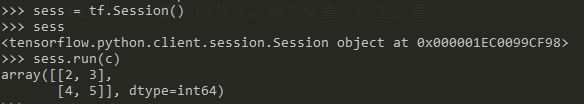mark
run(
fetches,
feed_dict=None,
options=None,
)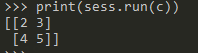mark

run返回的结果就是一个张量。

>>> tf.get_default_graph()
<tensorflow.python.framework.ops.Graph object at 0x000001EC0C5EE160>

>>> if c.graph is tf.get_default_graph():
...     print("The Graph of c is the default graph")
...
The Graph of c is the default graph


### 程序小例子

# -*- coding: UTF-8 -*-

# 引入tensorflow
import tensorflow as tf

# 设置了gpu加速提示信息太多了，设置日志等级屏蔽一些
import os
os.environ['TF_CPP_MIN_LOG_LEVEL']='2'

# 创建两个常量 Tensor.第一个为1行2列，第二个为二行一列。
# 也就是矩阵乘法必须满足，列等于行。
const1 = tf.constant([[2, 2]])
const2 = tf.constant([,
])

multiple = tf.matmul(const1, const2)

# 尝试用print输出multiple的值, 不会输出真实值。因为没运行
print(multiple)

# 创建了 Session (会话) 对象
sess = tf.Session()

# 用Session的run方法来实际运行multiple这个矩阵乘法操作
# 并把操作执行的结果赋值给 result
result = sess.run(multiple)

# 用print打印矩阵乘法的结果
print(result)

if const1.graph is tf.get_default_graph():
print("const1所在的图（Graph）是当前上下文默认的图")

# 关闭已用完的Session（会话）
sess.close()

# 第二种方法来创建和关闭Session,更安全
with tf.Session() as sess:
result2 = sess.run(multiple)
print("Multiple的结果是 %s " % result2)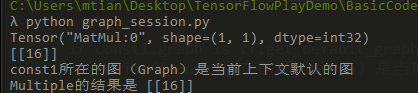mark

## 可视化利器Tensorboard

### 人工智能的黑盒mark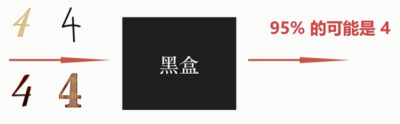markmarkmark

### TensorBoard的作用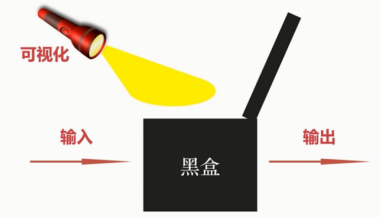mark

### 用TensorFlow保存图的信息到日志中


# 第一个参数为指定的保存路径，第二个参数为要保存的图
tf.summary.FileWriter("日志保存路径", sess.graph)


https://www.tensorflow.org/api_docs/python/tf/summary?hl=zh-cn

### 使用TensorBoard 读取并展示日志

tensorboard --logdir=日志所在路径


Tensorflow安装之后，会默认安装有TensorBoard

### Summary(总结，概览)

    # 第一个参数为指定的保存路径，第二个参数为要保存的图
tf.summary.FileWriter("./", sess.graph)

• 用于导出关于模型的精简信息的方法
• 可以使用TensorBoard等工具访问这些信息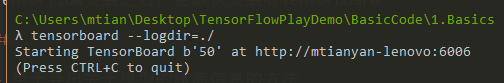mark

6006端口打开。mark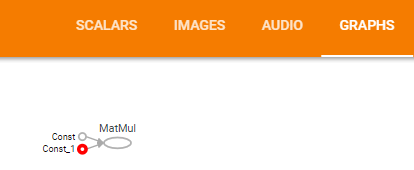mark

distributions 训练的一些分布。histograms 直方图。

### namespace(命名空间)

• 很像一些编程语言(如 c++) 的namespace， 包含嵌套的关系mark

### 符号的含义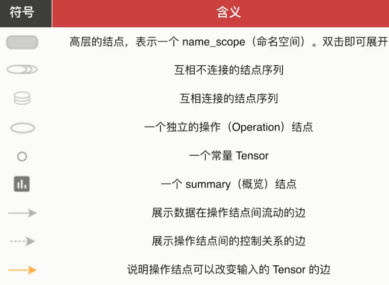mark

### 使用TensorBoard展示图代码示例

# -*- coding: UTF-8 -*-

# 引入tensorflow
import tensorflow as tf

# 设置了gpu加速提示信息太多了，设置日志等级屏蔽一些
import os
os.environ['TF_CPP_MIN_LOG_LEVEL']='3'

# 构造图(Graph)的结构
# 用一个线性方程的例子 y = W * x + b
# 梯度下降法求w和b
W = tf.Variable(2.0, dtype=tf.float32, name="Weight") # 权重
b = tf.Variable(1.0, dtype=tf.float32, name="Bias") # 偏差
x = tf.placeholder(dtype=tf.float32, name="Input") # 输入
with tf.name_scope("Output"):      # 输出的命名空间
y = W * x + b    # 输出

#const = tf.constant(2.0) # 常量，不需要初始化

# 定义保存日志的路径
path = "./log"

# 创建用于初始化所有变量 (Variable) 的操作
init = tf.global_variables_initializer()

# 创建Session（会话）
with tf.Session() as sess:
sess.run(init) # 初始化变量
# 写入日志文件
writer = tf.summary.FileWriter(path, sess.graph)
# 因为x是一个placeholder，需要进行值的填充
result = sess.run(y, {x: 3.0})
print("y = %s" % result) # 打印 y = W * x + b 的值，就是 7


tensorboard --logdir=./log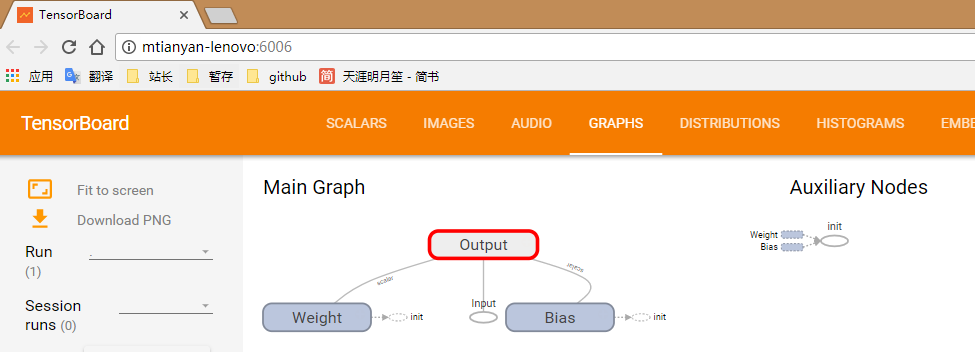mark

## 酷炫模拟游乐场playgroundmark

• JavaScript编写的网页应用
• 通过浏览器就可以训练简单的神经网络
• 训练过程可视化，高度定制化

https://playground.tensorflow.org/

epoch是完整的运行过程。

## 常用的python绘图库Matplotlibmark

https://matplotlib.org/

scipy下的一个组件。

• 很少的代码即可绘制2d 3d 静态动态等各种图形

• 一般常用的是它的子包: pyplot 提供类似matlab的绘图框架

### Matplotlib的一般绘图流程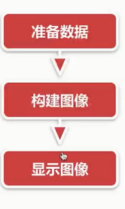mark
sudo pip install matplotlib


# -*- coding: UTF-8 -*-

# 引入 Matplotlib 的分模块 pyplot
import matplotlib.pyplot as plt
# 引入 numpy
import numpy as np

# 创建数据
# Linespace创建一定范围内的图线。-2到2之间等分100个点
x = np.linspace(-2, 2, 100)
#y = 3 * x + 4
y1 = 3 * x + 4
y2 = x ** 3

# 创建图像
#plt.plot(x, y)
plt.plot(x, y1)
plt.plot(x, y2)

# 显示图像
plt.show()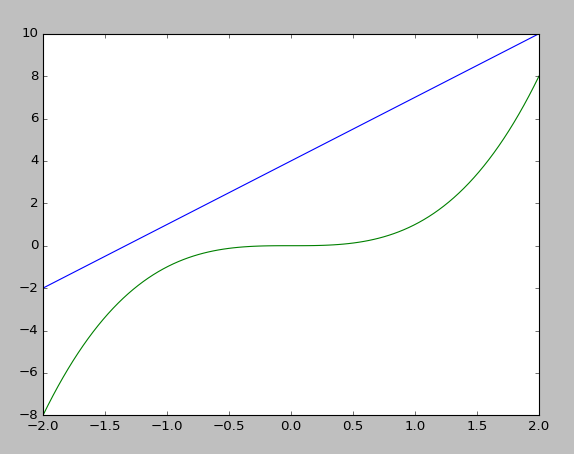mark

# -*- coding: UTF-8 -*-

import matplotlib.pyplot as plt
import numpy as np

# 创建数据
x = np.linspace(-4, 4, 50)
y1 = 3 * x + 2
y2 = x ** 2

# 第一张图
# 指定图的大小
plt.figure(num=1, figsize=(7, 6))
# 第一张图两个线
plt.plot(x, y1)
plt.plot(x, y2, color="red", linewidth=3.0, linestyle="--")

# 第二张图
plt.figure(num=2)
plt.plot(x, y2, color="green")

# 显示所有图像
plt.show()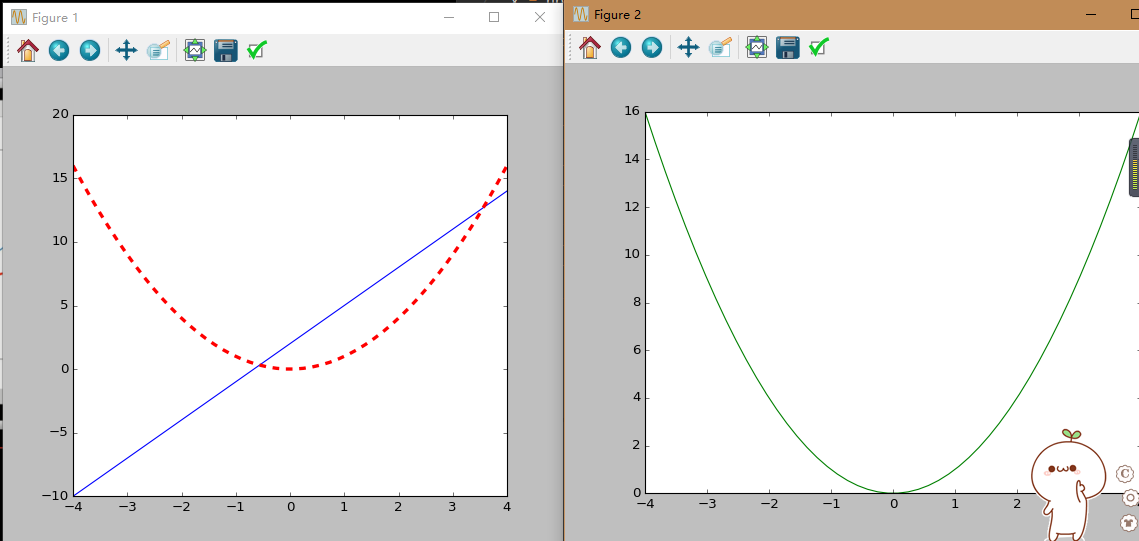mark

import numpy as np
import matplotlib.pyplot as plt

from matplotlib.ticker import NullFormatter  # useful for logit scale

# Fixing random state for reproducibility
# 为了重现结果，设置随机种子
np.random.seed(19680801)

# make up some data in the interval ]0, 1[
y = np.random.normal(loc=0.5, scale=0.4, size=1000)
y = y[(y > 0) & (y < 1)]
y.sort()
x = np.arange(len(y))

# plot with various axes scales
plt.figure(1)

# linear
# 两行两列子图中第一个
plt.subplot(221)
plt.plot(x, y)
plt.yscale('linear')
plt.title('linear')
plt.grid(True)

# log
plt.subplot(222)
plt.plot(x, y)
plt.yscale('log')
plt.title('log')
plt.grid(True)

# symmetric log
plt.subplot(223)
plt.plot(x, y - y.mean())
plt.yscale('symlog', linthreshy=0.01)
plt.title('symlog')
plt.grid(True)

# logit
plt.subplot(224)
plt.plot(x, y)
plt.yscale('logit')
plt.title('logit')
plt.grid(True)
# Format the minor tick labels of the y-axis into empty strings with
# NullFormatter, to avoid cumbering the axis with too many labels.
plt.gca().yaxis.set_minor_formatter(NullFormatter())
# Adjust the subplot layout, because the logit one may take more space
# than usual, due to y-tick labels like "1 - 10^{-3}"
wspace=0.35)

plt.show()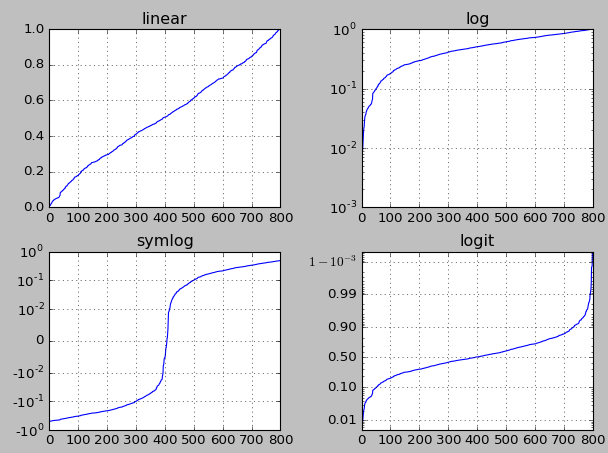mark

from mpl_toolkits.mplot3d.axes3d import Axes3D
import matplotlib.pyplot as plt
import numpy as np

fig, ax1 = plt.subplots(figsize=(8, 5),
subplot_kw={'projection': '3d'})

alpha = 0.8
r = np.linspace(-alpha,alpha,100)
X,Y= np.meshgrid(r,r)
l = 1./(1+np.exp(-(X**2+Y**2)))

ax1.plot_wireframe(X,Y,l)
ax1.plot_surface(X,Y,l, cmap=plt.get_cmap("rainbow"))
ax1.set_title("Bowl shape")

plt.show()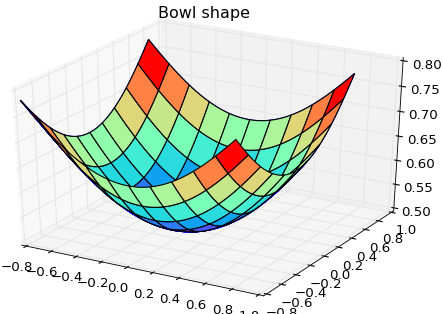mark

import numpy as np
from matplotlib import cm
import matplotlib.pyplot as plt
import mpl_toolkits.mplot3d.axes3d as p3
import matplotlib.animation as animation

def cost_function(x):
return x**2 + x**2

return np.array([2*x, 2*x])

nb_steps = 20
x0 = np.array([0.8, 0.8])
learning_rate = 0.1

def gen_line():
x = x0.copy()
data = np.empty((3, nb_steps+1))
data[:, 0] = np.concatenate((x, [cost_function(x)]))
for t in range(1, nb_steps+1):
data[:, t] = np.concatenate((x, [cost_function(x)]))
return data

def update_line(num, data, line):
# NOTE: there is no .set_data() for 3 dim data...
line.set_data(data[:2, :num])
line.set_3d_properties(data[2, :num])
return line

# Attaching 3D axis to the figure
fig = plt.figure()
ax = p3.Axes3D(fig)

# Plot cost surface
X = np.arange(-0.5, 1, 0.1)
Y = np.arange(-1, 1, 0.1)
X, Y = np.meshgrid(X, Y)
Z = cost_function((X, Y))

surf = ax.plot_surface(X, Y, Z, rstride=1, cstride=1, cmap=cm.coolwarm, linewidth=0, antialiased=False)

# Optimize
data = gen_line()

# Creating line objects
# NOTE: Can't pass empty arrays into 3d version of plot()
line = ax.plot(data[0, 0:1], data[0, 0:1], data[0, 0:1], 'rx-', linewidth=2)

# Setting the axes properties
ax.view_init(30, -160)

ax.set_xlim3d([-1.0, 1.0])
ax.set_xlabel('X')

ax.set_ylim3d([-1.0, 1.0])
ax.set_ylabel('Y')

ax.set_zlim3d([0.0, 2.0])
ax.set_zlabel('Z')

# Creating the Animation object
line_ani = animation.FuncAnimation(fig, update_line, nb_steps+1, fargs=(data, line), \
interval=200, blit=False)

plt.show()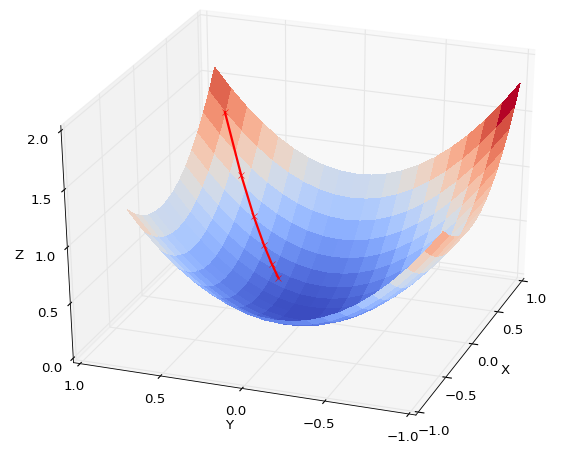mark

### tensorflow-mnist-tutorial的代码示例

https://github.com/martin-gorner/tensorflow-mnist-tutorial

ImportError: No module named 'tensorflowvisu'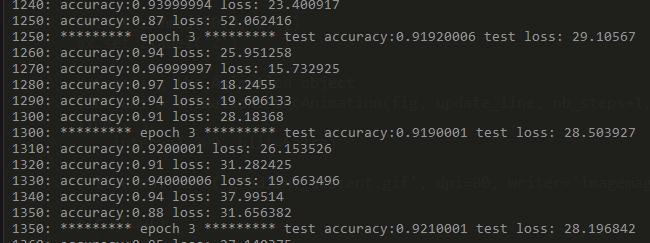mark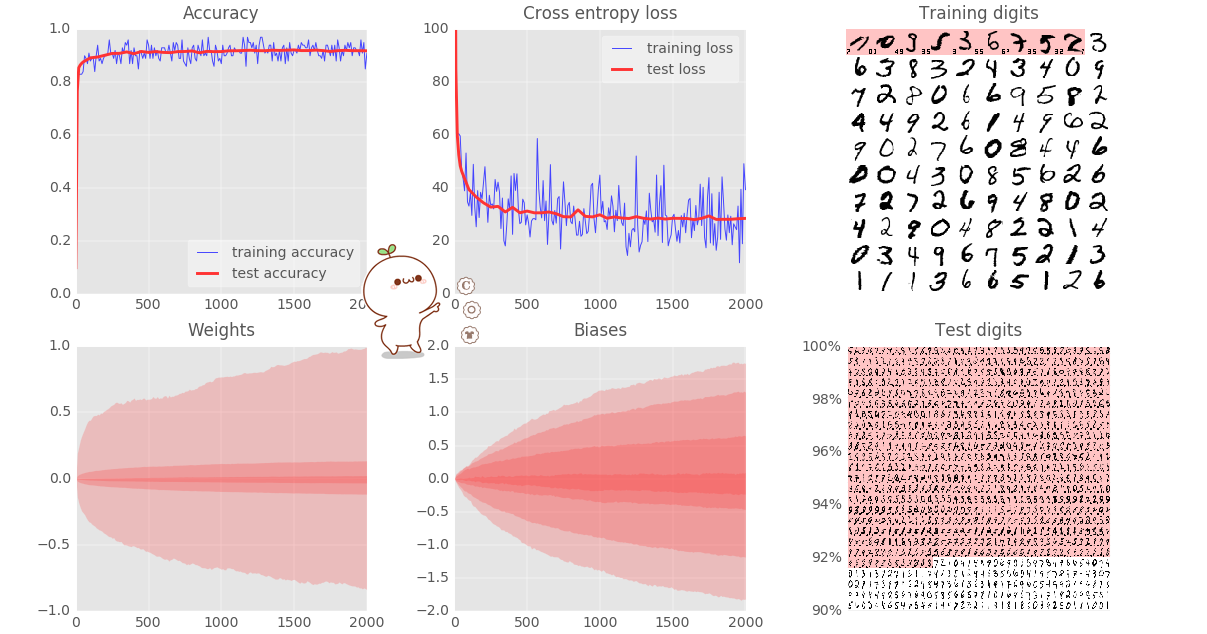mark

weights，权重。Biases，偏差。 测试的手写数字

## 综合小练习:梯度下降解决线性回归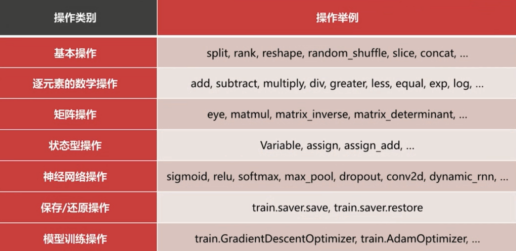mark
• 基本的操作:
• 逐元素的数学操作
• 矩阵操作
• 状态型操作
• 神经网络操作
• 保存，还原操作
• 模型训练操作
tf.nn.relu


### 一些等价的操作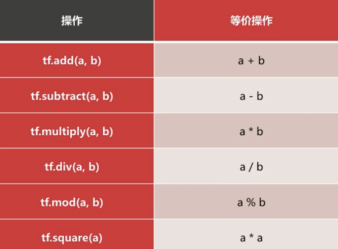mark

help(要查看的对象)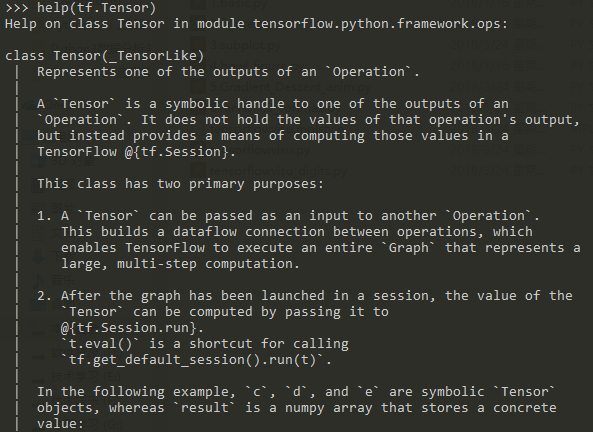mark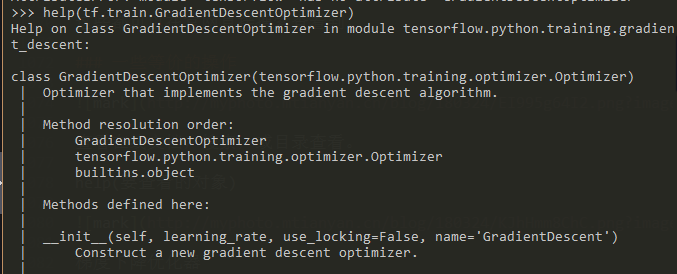mark

# -*- coding: UTF-8 -*-

'''

'''

import numpy as np
import matplotlib.pyplot as plt
import tensorflow as tf

# 构建数据：100个随机点
points_num = 100
# 之后要往vectors中填充100个点的值
vectors = []
# 用 Numpy 的正态随机分布函数生成 100 个点
# 这些点的(x, y)坐标值: 对应线性方程 y = 0.1 * x + 0.2
# 权重 (Weight) 为 0.1，偏差 (Bias)为 0.2
try:
# 运行100次
for i in xrange(points_num):
# 横坐标值，随机正态分布函数。区间0-0.66
x1 = np.random.normal(0.0, 0.66)
# 在真实值上加一些偏差
y1 = 0.1 * x1 + 0.2 + np.random.normal(0.0, 0.04)
# 将点list加入vectors列表中
vectors.append([x1, y1])
except:
for i in range(points_num):
x1 = np.random.normal(0.0, 0.66)
y1 = 0.1 * x1 + 0.2 + np.random.normal(0.0, 0.04)
vectors.append([x1, y1])

x_data = [v for v in vectors] # 列表生成式取出真实的点的 x 坐标
y_data = [v for v in vectors] # 真实的点的 y 坐标

# 图像 1 ：展示 100 个随机数据点
plt.plot(x_data, y_data, 'ro', label="Original data") # 红色圆圈圈型的点
# 展示label
plt.legend()
plt.show()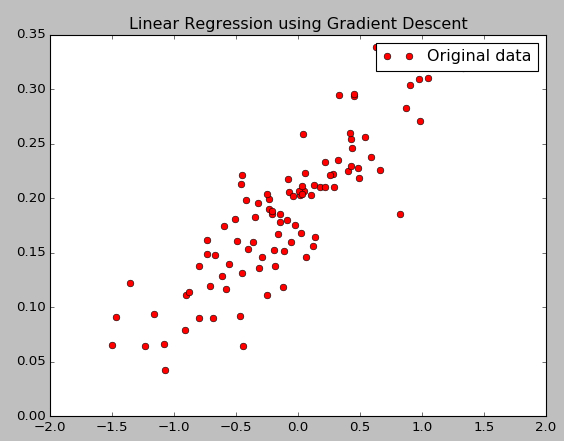mark

### 构建线性回归模型

# 构建线性回归模型

# 初始化参数，传入shape，最小值，最大值
W = tf.Variable(tf.random_uniform(, -1.0, 1.0)) # 初始化 Weight
# 偏差。初始化为0
b = tf.Variable(tf.zeros())                     # 初始化 Bias
# 这里的y是y帽子，也就是模型预测出来的值
y = W * x_data + b                                 # 模型计算出来的 y

# 定义 loss function (损失函数) 或 cost function (代价函数)
# 计算残差平方和。用(y帽子-真实的y)的平方累加的和。N就是总的点数，100.
# 对 Tensor 的所有维度计算 ((y - y_data) ^ 2) 之和 / N

# reduce_mean就是最后面的/N操作。square平方: y - y_data
loss = tf.reduce_mean(tf.square(y - y_data))

# 用梯度下降的优化器来优化我们的 loss functioin
# 让它更快的找到最终最拟合的w和b: 梯度下降的优化器。学习率，梯度下降的快慢。
optimizer = tf.train.GradientDescentOptimizer(0.5) # 设置学习率为 0.5(步长)，一般都是小于1的数。
# 太大的学习率可能错过局部最小值的那个点。
# 让它(损失函数)尽可能的损失最小
train = optimizer.minimize(loss)

# 创建会话
sess = tf.Session()

# 初始化数据流图中的所有变量
init = tf.global_variables_initializer()
sess.run(init)

try:
# 训练 20 步
for step in range(20):
# 优化每一步
sess.run(train)
# 打印出每一步的损失，权重和偏差.必须run才能得到实际的值。
print(("Step=%d, Loss=%f, [Weight=%f Bias=%f]") % (step, sess.run(loss), sess.run(W), sess.run(b)))
except:
# 训练 20 步
for step in xrange(20):
# 优化每一步
sess.run(train)
# 打印出每一步的损失，权重和偏差
print("Step=%d, Loss=%f, [Weight=%f Bias=%f]") \
% (step, sess.run(loss), sess.run(W), sess.run(b))

# 图像 2 ：绘制所有的点并且绘制出最佳拟合的直线
plt.plot(x_data, y_data, 'bo', label="Original data") # 蓝色圆圈的点
# 横坐标是x_data.纵坐标为此时的wb确定的y
plt.plot(x_data, sess.run(W) * x_data + sess.run(b), label="Fitted line") # 拟合的线
plt.legend()
plt.xlabel('x')
plt.ylabel('y')
plt.show()

# 关闭会话
sess.close()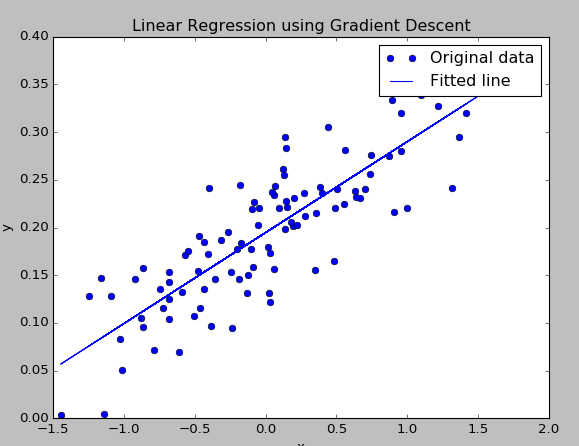mark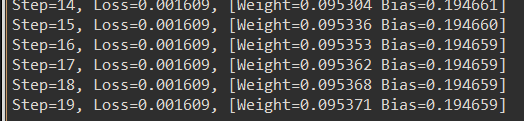mark

## 激活函数

tf.nn 点击nn

https://www.tensorflow.org/api_guides/python/nn#Activation_Functions

wiki中不同激活函数的图像，变种。

https://en.wikipedia.org/wiki/Activation_function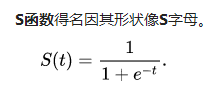mark
1 / float(1 + np.exp(-x))

# -*- coding: UTF-8 -*-

import numpy as np
import matplotlib.pyplot as plt
import tensorflow as tf

# 创建输入数据
x = np.linspace(-7, 7, 180) # (-7, 7) 之间等间隔的 180 个点

# 激活函数的原始手工实现
def sigmoid(inputs):
# y = 1 / (1 + exp(-x)) np.exp相当于e的多少次方
y = [1 / float(1 + np.exp(-x)) for x in inputs]
return y

def relu(inputs):
# f(x) = max(0,x) x大于0时，函数值y就是x。x<0时函数值y就是0.
# x如果大于0，则真值为1;y=x;而x若不满足>0真值为0;y=0
y = [x * (x > 0) for x in inputs]
return y

def tanh(inputs):
# e的x次方-e的负x次方做分母。e的x次方+e的负x次方做分母
y = [(np.exp(x) - np.exp(-x)) / float(np.exp(x) - np.exp(-x)) for x in inputs]
return y

def softplus(inputs):
# y = log(1+e的x平方)
y = [np.log(1 + np.exp(x)) for x in inputs]
return y

# 经过 TensorFlow 的激活函数处理的各个 Y 值
y_sigmoid = tf.nn.sigmoid(x)
y_relu = tf.nn.relu(x)
y_tanh = tf.nn.tanh(x)
y_softplus = tf.nn.softplus(x)

# 创建会话
sess = tf.Session()

# 运行run,得到四个返回值
y_sigmoid, y_relu, y_tanh, y_softplus = sess.run([y_sigmoid, y_relu, y_tanh, y_softplus])

# 创建各个激活函数的图像
plt.figure(1, figsize=(8, 6))

plt.subplot(221)
plt.plot(x, y_sigmoid, c='red', label='Sigmoid')
# y轴取值的区间
plt.ylim((-0.2, 1.2))
# 显示label，放在最适合的位置
plt.legend(loc='best')

plt.subplot(222)
plt.plot(x, y_relu, c='red', label='Relu')
plt.ylim((-1, 6))
plt.legend(loc='best')

plt.subplot(223)
plt.plot(x, y_tanh, c='red', label='Tanh')
plt.ylim((-1.3, 1.3))
plt.legend(loc='best')

plt.subplot(224)
plt.plot(x, y_softplus, c='red', label='Softplus')
plt.ylim((-1, 6))
plt.legend(loc='best')

# 显示图像
plt.show()

# 关闭会话
sess.close()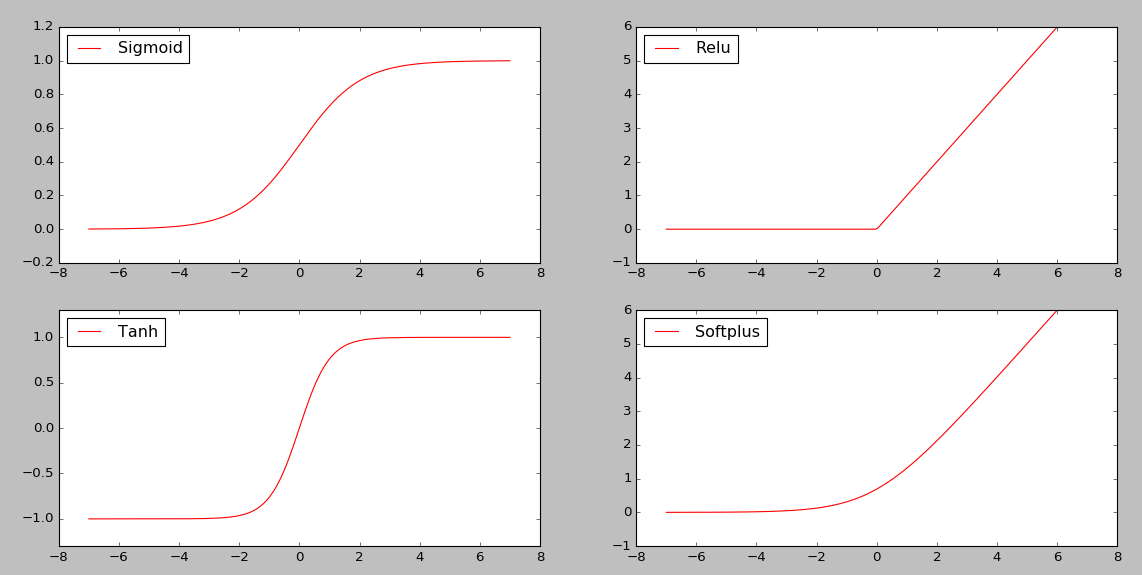mark

## 动手实现cnn卷积神经网络

TensorFlow中封装了这个数据集。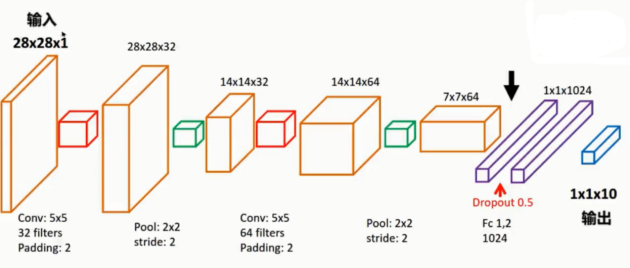mark

# -*- coding: UTF-8 -*-

import numpy as np
import tensorflow as tf

# 下载并载入 MNIST 手写数字库（55000 * 28 * 28）55000 张训练图像
from tensorflow.examples.tutorials.mnist import input_data

# one_hot 独热码的编码 (encoding) 形式
# 0, 1, 2, 3, 4, 5, 6, 7, 8, 9 的十位数字
# 第一位上被激活，唯一的表示这一个数字。
# 0 : 1000000000
# 1 : 0100000000
# 2 : 0010000000
# 3 : 0001000000
# 4 : 0000100000
# 5 : 0000010000
# 6 : 0000001000
# 7 : 0000000100
# 8 : 0000000010
# 9 : 0000000001

# onehot设置True会表示成onehot的编码。否则会表示本身。

# None 表示张量 (Tensor) 的第一个维度可以是任何长度 除以255
input_x = tf.placeholder(tf.float32, [None, 28 * 28]) / 255. # 输入
output_y = tf.placeholder(tf.int32, [None, 10]) # 输出：10个数字的标签
input_x_images = tf.reshape(input_x, [-1, 28, 28, 1]) # 改变形状之后的输入


## 理清卷积神经网络的原理和思路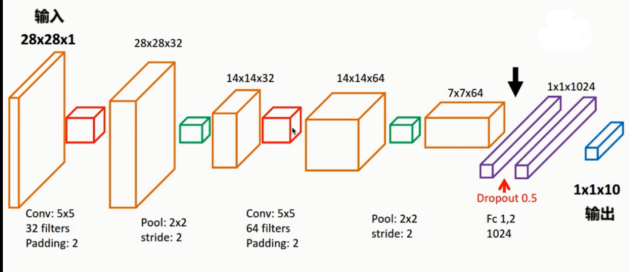mark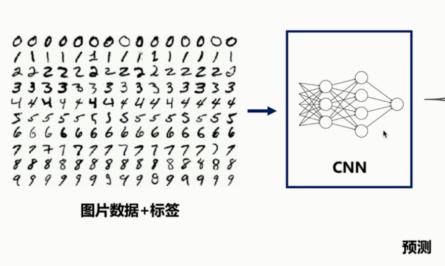mark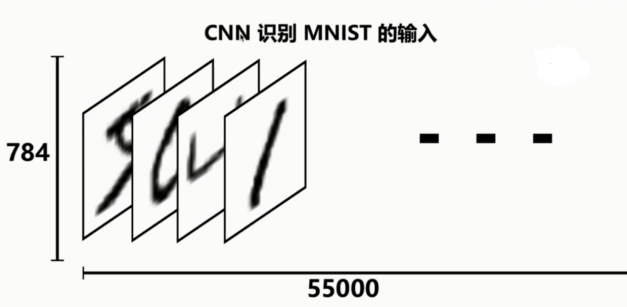mark

28,28像素的一个图片。784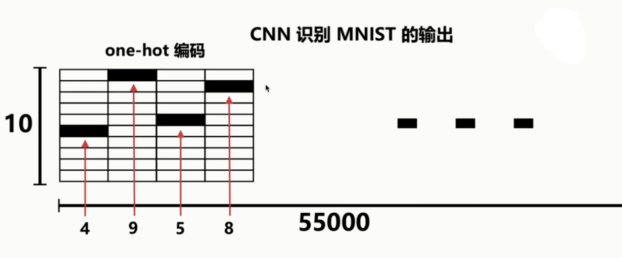mark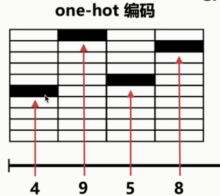mark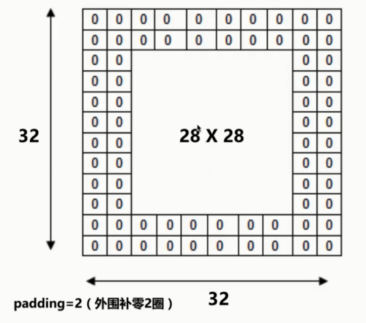mark

# 从 Test（测试）数据集里选取 3000 个手写数字的图片和对应标签
test_x = mnist.test.images[:3000] # 图片
test_y = mnist.test.labels[:3000] # 标签

# 构建我们的卷积神经网络：
# 第 1 层卷积(二维的卷积) tf.nn 和 tf.layers 中的cov2d有相似有不同。
# 让图像经过卷积层，维度变为28*28*32。用一个5,5的过滤器(采集器)。
# 从左上角到右下角一点一点采集。每个过滤器扫一遍，输出增加一层。
# 扫了32遍，深度就会从1变为32
# 第二个卷积层，扫了64遍，变成了64

# tf.layers.conv2d二维的卷积函数(https://www.tensorflow.org/api_docs/python/tf/layers/conv2d?hl=zh-cn)
conv1 = tf.layers.conv2d(
inputs=input_x_images, # 形状 [28, 28, 1]，这里还是一个placeholder，后面会填充值
filters=32,            # 32 个过滤器，输出的深度（depth）是32
kernel_size=[5, 5],    # 过滤器(卷积核心)在二维的大小是(5 * 5)
strides=1,             # 卷积步幅,步长是1
padding='same',        # same 表示输出的大小不变，因此需要在外围补零 2 圈
activation=tf.nn.relu  # 激活函数是 Relu
) # 形状 [28, 28, 32]


# 第 1 层池化（亚采样）比原来的那些数据，输出没有输入那么多、
# 只采一部分数据。
pool1 = tf.layers.max_pooling2d(
inputs=conv1,     # 形状 [28, 28, 32]
pool_size=[2, 2], # 过滤器在二维的大小是（2 * 2）
strides=2         # 步长是 2
) # 经过亚采样之后，形状 [14, 14, 32]


pooling有几种方案。平均，max。平面区域中选最大的值。

https://www.tensorflow.org/api_docs/python/tf/layers/max_pooling2d?hl=zh-cn

### 构建第二层卷积

# 第 2 层卷积
conv2 = tf.layers.conv2d(
inputs=pool1,          # 输入形状 [14, 14, 32]
filters=64,            # 64 个过滤器，输出的深度（depth）是64
kernel_size=[5, 5],    # 过滤器在二维的大小是(5 * 5)
strides=1,             # 步长是1
padding='same',        # same 表示输出的大小不变，因此需要在外围补零 2 圈
activation=tf.nn.relu  # 激活函数是 Relu
) # 输出形状 [14, 14, 64]


### 构建第二层池化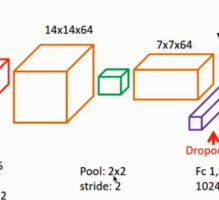mark

# 第 2 层池化（亚采样）
pool2 = tf.layers.max_pooling2d(
inputs=conv2,     # 形状 [14, 14, 64]
pool_size=[2, 2], # 过滤器在二维的大小是（2 * 2）
strides=2         # 步长是 2
) # 输出形状 [7, 7, 64]


### flat平坦化

# 平坦化（flat）
flat = tf.reshape(pool2, [-1, 7 * 7 * 64]) # 形状 会变成[7 * 7 * 64, ]


### 全连接层

# 1024 个神经元的全连接层
dense = tf.layers.dense(inputs=flat, units=1024, activation=tf.nn.relu)


### Dropout

# Dropout : 丢弃 50%, rate=0.5
dropout = tf.layers.dropout(inputs=dense, rate=0.5)


### 再经过一个10个神经元的全连接层，也就是真实的输出了。

# 10 个神经元的全连接层，这里不用激活函数来做非线性化了
logits = tf.layers.dense(inputs=dropout, units=10) # 输出。形状[1, 1, 10]


### 计算误差

# 计算误差 (计算 Cross entropy (交叉熵)，再用 Softmax 计算百分比概率)
loss = tf.losses.softmax_cross_entropy(onehot_labels=output_y, logits=logits)


https://www.tensorflow.org/api_docs/python/tf/losses/softmax_cross_entropy

onehot_labels 就是我们真实的标签值，output_y，暂时是一个placeholder，之后会赋值(训练集里对应的真实标签)。

logits 传入我们的预估，logits(y帽子)

# Adam 优化器来最小化误差，学习率 0.001使之最小化。使loss最小


### 精度，计算预测值 和 实际标签的匹配程度

# 精度。计算 预测值 和 实际标签 的匹配程度
# 返回(accuracy, update_op), 会创建两个局部变量
accuracy = tf.metrics.accuracy(
labels=tf.argmax(output_y, axis=1),
predictions=tf.argmax(logits, axis=1),)


https://www.tensorflow.org/api_docs/python/tf/metrics/accuracy

Calculates how often predictions matches labels

argmax函数会返回张量轴上最大值的下标。

accuracy函数会返回两个值，我们取其中一个值。

accuracy：Tensor代表准确度，total除以的值count。
update_op：一种适当增加total和count变量并且其值匹配的操作accuracy。


## 训练神经网络

# 创建会话
sess = tf.Session()

# 初始化变量：全局和局部
init = tf.group(tf.global_variables_initializer(), tf.local_variables_initializer())
sess.run(init)


https://www.tensorflow.org/api_docs/python/tf/group

创建一个将多个操作分组的操作。


### 训练我们的神经网络

# 训练神经网络
for i in range(20000):
# 从训练集中进行选取。batch，一包。
batch = mnist.train.next_batch(50)  # 从 Train（训练）数据集里取"下一个"50 个样本
# run之后，loss的返回值给到train_loss train_op的值给到train_op_
# 给实际的input_x和input_y赋值。batch有两列，0是图片，1是真实标签。
train_loss, train_op_ = sess.run([loss, train_op], {input_x: batch, output_y: batch})
if i % 100 == 0:
# 这里测试的精度是在测试集上的精度。
test_accuracy = sess.run(accuracy, {input_x: test_x, output_y: test_y})
# 步数，训练的损失，测试的精度
print(("Step=%d, Train loss=%.4f, [Test accuracy=%.2f]") \
% (i, train_loss, test_accuracy))


### 测试，打印20个预测值和真实值的对

# 测试：打印 20 个预测值 和 真实值 的对
# 输入测试集的20项，输出预测的y(onehot)
test_output = sess.run(logits, {input_x: test_x[:20]})
# 取到它预测的y是哪个真实数字
inferenced_y = np.argmax(test_output, 1)
print(inferenced_y, 'Inferenced numbers') # 推测的数字

# 取出测试集中的真实标签值
print(np.argmax(test_y[:20], 1), 'Real numbers') # 真实的数字


### 训练结果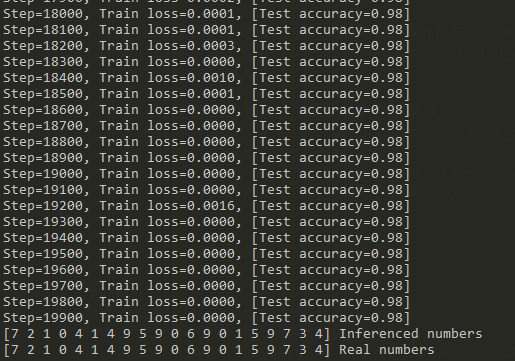mark

#### 教你编写人工智能程序教程（自学必看）

05-0502-115473

#### AI人工智能 Python实现人机对话

04-091万+

#### 人工智能---图像识别

03-21674

#### 最新《Python人工智能学习启蒙教程》

05-311055

#### 人工智能入门基础python编程

07-271132

#### AI人工智能-Python实现人机对话

12-255048

#### 大家Python写好的AI程序怎么做出界面？

11-05

07-263020

#### 10个人工智能Python项目，赶紧收藏!!!©️2020 CSDN 皮肤主题: 大白 设计师: CSDN官方博客点击重新获取扫码支付1.余额是钱包充值的虚拟货币，按照1:1的比例进行支付金额的抵扣。
2.余额无法直接购买下载，可以购买VIP、C币套餐、付费专栏及课程。余额充值